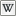# LlAtan2

(diff) ← Older revision | Latest revision (diff) | Newer revision → (diff)

 LSL Portal
Function: float llAtan2( float y, float x );
 3 Function ID 0 Forced Delay 10 Energy

Returns a float that is thearctangent2 of y, x.

 • float y • float x

Similar to the (y/x) except it utilizes the signs of x & y to determine the quadrant and avoids division by zero.

## Specification

If x is positive zero and...

• y is zero, zero is returned.
• y is positive, PI/2 is returned.
• y is negative, -PI/2 is returned.

If x is negative zero and...

• y is positive zero, PI is returned.
• y is negative zero, -PI is returned.
• y is positive, PI/2 is returned.
• y is negative, -PI/2 is returned.

Or

```if((string)x != (string)0.0 && y == 0.0)//negative zero
return PI * ~-2*((string)y != (string)0.0));
return ((y > 0) - (y < 0)) * PI_BY_TWO;
```

The returned value is in the range [-PI, PI].

## Examples

```default
{
state_entry()
{
float num1 = llFrand(100.0);
float num2 = llFrand(100.0);

llOwnerSay("y = " + (string)num1);
llOwnerSay("x = " + (string)num2);

llOwnerSay("The arctangent of y divided by x is " + (string)llAtan2(num1, num2));
}
}
```
```//Function with input of a vector determining the position of a target and returning
//a string with the literal compass-direction of that target towards your position
//by Ramana Sweetwater 2009/01, any use allowed license :-)
//corrected by Patrick Muggins

string compass (vector target)
{
vector source = llGetPos();
list DIRS =["W","NW","N","NE","E","SE","S","SW","W"];
integer index = llCeil(3.5 - (4 * llAtan2(target.y - source.y, target.x - source.x) / PI));
return llList2String(DIRS, index);
}
```
/Compass QA

### Functions

 • llSin llAsin – sine & inverse Sine • llCos llAcos – cosine & inverse cosine • llTan – tangent

## Deep Notes

Search JIRA for related Issues

#### Footnotes

1. ^ The ranges in this article are written in Interval Notation.

#### Signature

```function float llAtan2( float y, float x );
```

#### Haiku

An analog clock
passes through infinity
twice every single hour